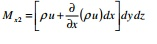Home | | Mechanics of Fluids | Fluid Kinematics And Dynamics: Continuity Equation

# Fluid Kinematics And Dynamics: Continuity EquationThe derivation is based on the concept of Law of conservation of mass. Statement: The flow of fluid in a continuous flow across a section is always a constant.

Continuity Equation

The derivation is based on the concept of Law of conservation of mass.

Statement: The flow of fluid in a continuous flow across a section is always a constant.

Consider an enlarging section in a fluid flow of fluid density?. Consider two sections 1 and 2 as shown in Fig. Let the sectional properties be as under

A1and A2 = Cross-sectional area, V1 and V2 = Average flow velocity and ?1 and ?2 = Fluid density at Sec 1 and 2 respectively

dt is the time taken for the fluid to cover a distance dx The mass of fluid flowing across section 1-1 is given by

m1  = Density at section 1 x volume of fluid that has crossed section 1

?1  x A1  x dx

Mass rate of fluid flowing across section 1-1 is given by

m1/dt = (Density at sec 1 x volume of fluid that has crossed sec 1)/dt

?1  x A1  x dx/dt = ?1 A1V1 �(01)

Similarly Mass rate of fluid flowing across section 2-2 is given by

m2/dt = ?2  x A2  x dx/dt = ?2A2 V2 �(02)

From law of conservation of mass, mass can neither be created nor destroyed.Hence from Eqs. 1 and 2, we get

?1A1 V1  = ?2 A2V2

If the density of the fluid is constant, then the equation reduces to

A1V1  = A2 V2

The above equation is discharge continuity equation in one dimensional form for an steady, incompressible fluid flow

Continuity Equation in three dimensional or differential forms

Consider a parallelepiped ABCDEFGH in a fluid flow of density ? as shown in Fig. Let the dimensions of the parallelepiped be dx, dy and dz along x, y and z directions respectively. Let the velocity components along x, y and z be u, v and w respectively.

Similarly mass rate of fluid flow leaving the section EFGH along x direction is given byNet gain in mass rate of the fluid along the x axis is given by the difference between the mass rate of flow entering and leaving the control volume. i.e. Eq. 1 - Eq. 2This expression is known as the general Equation of Continuity in three dimensional form or differential form.

If the fluid is incompressible then the density is constant and henceThe continuity equation in two-dimensional form for compressible and incompressible flows are respectively as belowStudy Material, Lecturing Notes, Assignment, Reference, Wiki description explanation, brief detail
Civil - Mechanics Of Fluids - Fluid Kinematics And Dynamics : Fluid Kinematics And Dynamics: Continuity Equation |

Related Topics